# for循环、while循环，字符串变量的相关总结。彩世

## PYTHON之路(第二篇)：PYTHON基本数据类型，python第二篇

1、编码
UTF-8:中文占3个字节
GBK：中文占2个字节
Unicode、UTF-8、GBK三者关系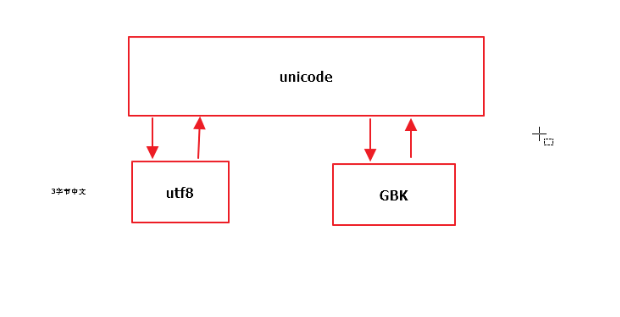2、input（）函数

``````n = input（" "）
>>>hello
>>>n
>>>'hello'
``````

``````n = input（" "）
>>>10
>>>n
>>>'10'
``````

n * 10将输出
'10101010101010101010'

new_n = int(n)

3、while循环、continue、break

``````while 条件语句1：
功能代码1
else 条件语句2：
功能代码2
``````

while循环也可以加else

``````n = 1
while n < 11:
if n == 7 :
pass
else:
print(n)
n = n   1
``````

``````count = 1
while count < 11
if count == 7:
count = count   1
continue
print(count)
count = count   1
``````

• 1不会被执行，重新跳回while语句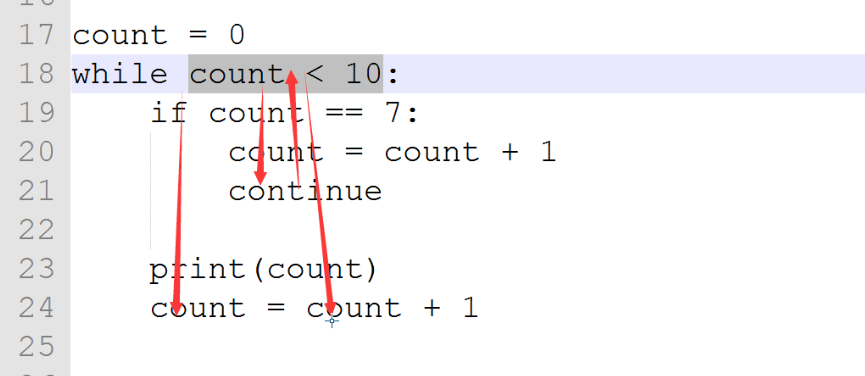``````count = 1
while count < 11:
count = count   1
continue
print('123')
print('end')
``````

``````count = 1
while count < 11:
count = count   1
print(count)
break
print('123')
print('end')
``````

``````2
end
``````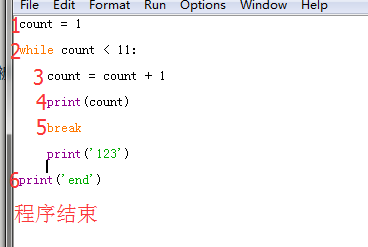4、算术运算符
-     *     /      %        **         //

5、字符串

``````name = "马大帅"
if "马" in name :
print("ok")
else:
print("error")
``````

'马大帅' 称为字符串
'马' 成为一个字符
'马大'或者'大帅'称为子字符串，也可以叫做子序列，注意这里的字符要连续的，而'马帅'不能称之为子字符串

6、成员运算：

``````name = "马大帅"
if "吗" not in name :
print("ok")
else:
print("error")
``````

7、布尔值

if语句和while语句都使用布尔值作为条件。

``````if 条件判断语句
功能代码某块
``````

``````name = "马大帅"
p ="吗" not in name
print(p)
if p:
print("ok")
else:
print("error")
``````

``````True
ok
``````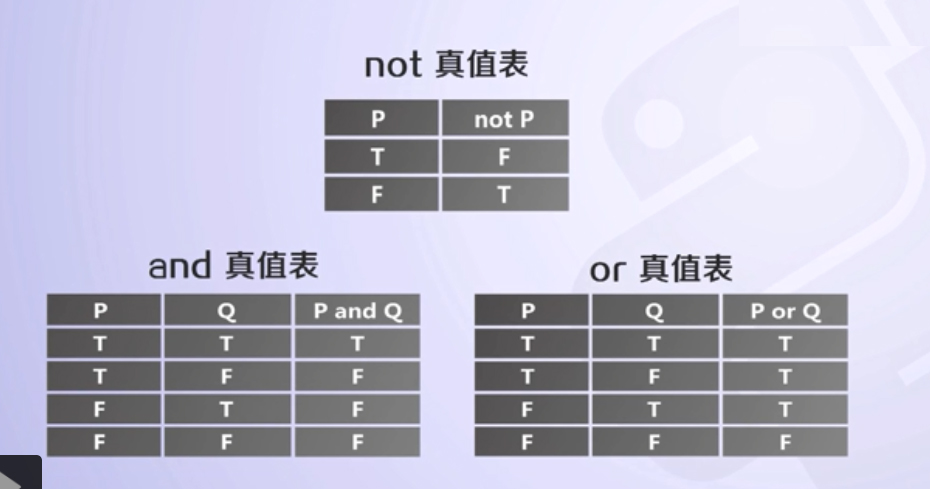``````i = 10
while i:
print ("我爱学习！")
print("end")
``````

``````我爱学习！

...（这里代表一直输出"我爱学习"）
``````

``````i = 10
while i:
print ("我爱学习！",i)
i = i -1
print("end")
``````

``````我爱学习！ 10

end
``````

print("end")语句。

8、比较运算符：判断大小符号
== 等于
> 大于
< 小于
>= 大于等于
<= 小于等于
！= 不等于

9、运算的优先级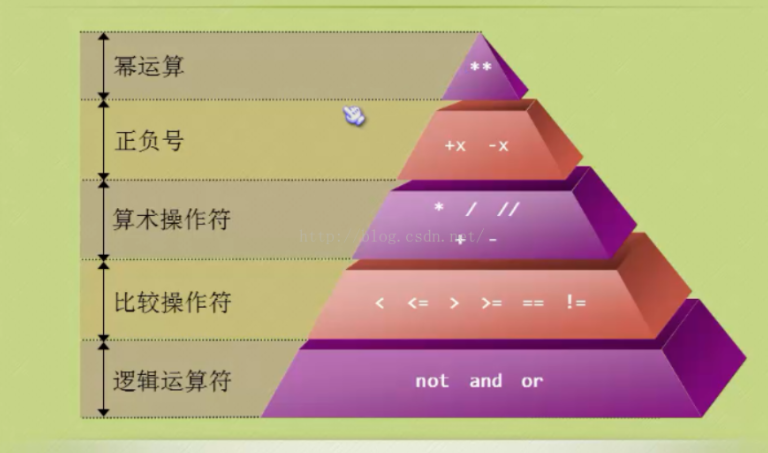``````user = 'nicholas'
psswd ='123'
v = user == 'nicholas' and passwd == '123' or 1 == 2 and pwd == '9876'
print(v)
``````

v = true and true or

(1)第一个表达式 or
True or ————>>得出结果True
(2)第一个表达式 and
True and ————>>继续运算
（3）第一个表达式 or
False or ————>>继续运算
（4）第一个表达式 and
False and ————>>得出结果False

or 左侧表达式 。

表达式从左至右运算，若 and 的左侧逻辑值为 False ，则短路其后所有 and 表达式，直到有 or 出现，输出 and 左侧表达式到
or 的左侧，参与接下来的逻辑运算。

若 or 的左侧为 False ，或者 and 的左侧为 True 则不能使用短路逻辑。

10、赋值运算符
>= 简单的赋值运算符 c = a b 将 a b 的运算结果赋值为 c
= 加法赋值运算符 c = a 等效于 c = c a
-= 减法赋值运算符 c -= a 等效于 c = c - a
*= 乘法赋值运算符 c *= a 等效于 c = c * a
/= 除法赋值运算符 c /= a 等效于 c = c / a
%= 取模赋值运算符 c %= a 等效于 c = c % a
**= 幂赋值运算符 c **= a 等效于 c = c ** a
//= 取整除赋值运算符 c //= a 等效于 c = c // a

(1)数字 int
a = 1
a = 2

int整型（整数类型）
python3中用int表示，没有范围
python2中int有一定范围

python3中只有整型，用int，取消了long类型

**①**、int()将字符串转换为数字

``````a = "123"
type(a)
b = int(a)
print(b)
type(b)
``````

``````<class 'str'>
123
<class 'int'>
``````

type（）即可查看变量类型

a = "123n"
b = int(a)

``````num = "c"
v = int(num,base = 16)
print(v)
``````

②bit_lenght

``````age = 5
r = age.bit_length()
#当前数字的二进制，至少占用了n位表示
print(r)
``````

输出结果

``````3
``````

即5在二进制中表示为101，至少需要3个位置来表示

（2）字符串 str
a ='hello'
a= 'ssssdda'

a--capitalize()

``````# capitalize() 首字母大写
test = "lingou"
v1 = test.capitalize( )
print(v1)
``````

``````Lingou
``````

b--casefold( )、lower()

#lower() 所有变小写
# casefold( ) 所有变小写，与lower相比casefold更牛逼，很多未知(不是英文的，如法文、德文等)的对相应变小写

#lower() 方法只对ASCII编码，也就是‘A-Z’有效，对于其他语言（非汉语或英文）中把大写转换为小写的情况只能用 casefold() 方法。

``````test = "LinGou"
v2 = test.casefold( )
print(v2)
v3 =test.lower()
print(v3)
``````

输出结果

``````lingou
lingou
``````

c--center( )

#center( ) 设置宽度，并将内容居中,这里的"*"可以不写，默认为空白。
#这里的30是总宽度，单位字节

``````test = "LinGou"
v4 = test.center(30,"*" )
print(v4)
``````

``````************LinGou************
``````

``````test = "LinGou"
v5 = test.center(30 )
print(v5)
``````

``````          LinGou
``````

d--count( )

#count( ) 去字符串中寻找，寻找子序列的出现次数

#count(sub[, start[, end]])

#count( 子序列,寻找的开始位置，寻找的结束位置)

#count( sub, start=None, end=None) None默认表示此参数不存在

``````test = "LinGouLinGengxin"
v6 = test.count("in" )
print(v6)
v7 = test.count("in",3,6)
#这里的3，6 是对字符串"LinGouLinGengxin"的索引编码，从第三个开始到第六个结束
#L i n G o u L i n G  e  n  g  x  i  n
#0 1 2 3 4 5 6 7 8 9 10 11 12 13 14 15
print(v7)
v8 = test.count("in",3)#从第3个位置开始找
print(v8)
``````

``````3
0
2
``````

e--endswith（）、startswith（）

#endswith（） 以什么什么结尾

#startswith（）以什么什么开始

``````test = "LinGouLinGengxin"
v9 = test.endswith("in" )
v10 = test.startswith("in")
print(v9)
print(v10)
``````

``````True
False
``````

f--find（）、index（）

#find（）从开始往后找，找到第一个之后，获取其索引位置
#index（）功能同上，index找不到，报错,一般建议用find()

``````test = "LinGouLinGengxin"
v11 = test.find("in" )
v12 = test.find("XING" )
v13 = test.index("in")
# v14 = test.index("XING" )
print(v11)
print(v12)
print(v13)
#print(v14)
``````

``````1
-1
1
``````

g--format（）

#format（）格式化，将一个字符串中的占位符替换为指定的值
# { }就是占位符，通过format将占位符替换为指定的值

``````test = "I am {name}"
print(test)
v15 = test.format(name = "LinGou" )
print(v15)
``````

``````I am {name}
I am LinGou
``````

-第二个

``````test = "I am {name},age{a}"
print(test)
v16 = test.format(name = "LinGou",a = 19 )
print(v16)
``````

``````I am {name},age{a}
I am LinGou,age19
``````

-第三个

``````test = "I am {0},age{1}"
print(test)
v17 = test.format("LinGou",19 )
print(v17)
``````

``````I am {0},age{1}
I am LinGou,age19
``````

#format_map（）格式化，传入的值
# 书写格式{"name":"LinGou","a":19}

``````test = "I am {name},age {a}"
print(test)
v18 = test.format_map({"name":"LinGou","a":19} )
v19 = test.format(name = "LinGou",a = "19")
print(v18)
print(v19)
``````

``````I am {name},age {a}
I am LinGou,age 19
I am LinGou,age 19
``````

f--isalnum( )

#isalnum( )字符串中是否只包含 字母和数字

``````test = "LinGou"
v20 = test.isalnum( )
print(v20)
test2 = "LinGou "
v21 = test2.isalnum( )
print(v21)
``````

``````True
False
``````

#### 1.赋值运算符

* 在 Python 中，使用 = 可以给变量赋值

* 在算术运算时，为了简化代码的编写，Python 还提供了一系列的 与 算术运算符 对应的 赋值运算符

* 注意：赋值运算符中间不能使用空格

=

9、运算的优先级

### 5.字符串。

print（name【1，3】）结果为bc

#### 删除

del 列表[索引]：删除指定索引的数据

``````I am {name},age {a}
I am LinGou,age 19
I am LinGou,age 19
``````

### 2.索引切片。### 3.字符串常见的操作:

1.find （） 检测所要搜索得字符是否包含在oldstr中，

2.index（）和find大体是一样的。index错误会显示报错，而find报的是-1.

3.count（）返回newstr在start和end之间oldstr出现的次数。

4.replace（）把oldstr中newstr1替换成newstr2，如果count指定，则替换不超过count次

#### 简单的赋值运算符

c = a b 将 a b 的运算结果赋值为 c

=

c = a 等效于 c = c a

-=

c -= a 等效于 c = c - a

*=

c = a 等效于 c = c a

/=

c /= a 等效于 c = c / a

//=

c //= a 等效于 c = c // a

%=

c %= a 等效于 c = c % a

**=

c = a 等效于 c = c a

* 自然计数法（从 1 开始）—— 更符合人类的习惯

* 程序计数法（从 0 开始）—— 几乎所有的程序语言都选择从 0 开始计数

*

#### 拆分和连接

string.partition(str)

string.rpartition(str)

string.split(str=”“, num)

string.splitlines()

string.join(seq)

#### 统计

len(列表)：列表长度

``````a = "123"
type(a)
b = int(a)
print(b)
type(b)
``````

### 二、for循环

for循环 ：  把变量一个一个拿出来。

``````           LinGou
``````

#### 4.break与continue区别。

i=0

while 1：

print（‘这是第%d次循环’%i）

i = 1

if i > 5：

break

for i in range (0,5):

num = int(input('请输出你本次抓娃娃需要多少秒（1-60秒）‘)

if num >30:

print('时间到了，机器自动抓给你啦')

else：

print（’你本次用了%d秒抓了一下‘num）

num = int(input('请输入你本次抓娃娃需要多少秒(1~60秒)'))

if num > 30:

print("时间到啦，机器自动给你抓了")

continue

print('你本次用了%d秒抓了一下'%num)

ascii和unicode可以相互转换，gbk和unicode可以相互转换。

### 1.字符串下标的使用。

``````test = "LinGou"
v20 = test.isalnum( )
print(v20)
test2 = "LinGou "
v21 = test2.isalnum( )
print(v21)
``````

#### 大小写转换 -

string.capitalize()

string.title()

string.lower()

string.upper()

string.swapcase()

(1)第一个表达式 or
True or ————>>得出结果True
(2)第一个表达式 and
True and ————>>继续运算
（3）第一个表达式 or
False or ————>>继续运算
（4）第一个表达式 and
False and ————>>得出结果False

#### 1.for循环的格式：for 循环变量 in 对象：

print（i）

#count(sub[, start[, end]])

### continue是忽略单次操作，剩下的正常运行。

表达式从左至右运算，若 and 的左侧逻辑值为 False ，则短路其后所有 and 表达式，直到有 or 出现，输出 and 左侧表达式到
or 的左侧，参与接下来的逻辑运算。

#### 2.for经常和range连用

for i in range（0，10，2）

print（i）

#### 3.break和continue在for循环中也可以用。

break是终止循环。

``````i = 10
while i:
print ("我爱学习！")
print("end")
``````

## 三、字符串及高级变量类型的使用。

or 左侧表达式 （即True）。

### 一、while循环

#### 字典

* 列表 是 有序 的对象集合* 字典 是 无序 的对象集合

* 字典用 {} 定义

* 字典使用 键值对 存储数据，键值对之间使用 , 分隔

* 键 key 是索引

* 值 value 是数据

* 键 和 值 之间使用 : 分隔

* 键必须是唯一的

* 值 可以取任何数据类型，但 键 只能使用 字符串、数字或 元组

10、赋值运算符
>= 简单的赋值运算符 c = a b 将 a b 的运算结果赋值给c
= 加法赋值运算符 c = a 等效于 c = c a
-= 减法赋值运算符 c -= a 等效于 c = c - a
*= 乘法赋值运算符 c *= a 等效于 c = c * a
/= 除法赋值运算符 c /= a 等效于 c = c / a
%= 取模赋值运算符 c %= a 等效于 c = c % a
**= 幂赋值运算符 c **= a 等效于 c = c ** a
//= 取整除赋值运算符 c //= a 等效于 c = c // a

#### 3.continue

* 在循环过程中，如果 某一个条件满足后，不 希望 执行循环代码，但是又不希望退出循环，可以使用 continue

* 也就是：在整个循环中，只有某些条件，不需要执行循环代码，而其他条件都需要执行

``````我爱学习！

...（这里代表一直输出"我爱学习"）
``````

#### 4.字符串中的转义字符

* t 在控制台输出一个 制表符，协助在输出文本时 垂直方向 保持对齐

* n 在控制台输出一个 换行符

\

n

t

r回车

#### 文本对齐 -

string.ljust(width)

string.rjust(width)

string.center(width)

• 3

string.lstrip()

string.rstrip()

string.strip()

-第三个

#### 查找和替换 -

string.startswith(str)

string.endswith(str)

string.find(str, start=0, end=len(string))

string.rfind(str, start=0, end=len(string))

string.index(str, start=0, end=len(string))

string.rindex(str, start=0, end=len(string))

string.replace(old_str, new_str, num=string.count(old))

注：这个输出结果LinGou左右是有空格的

#### 字符串.

string.isspace()：如果 string 中只包含空格，则返回 True

string.isalnum()：如果 string 至少有一个字符并且所有字符都是字母或数字则返回 True

string.isalpha()：如果 string 至少有一个字符并且所有字符都是字母则返回 True

string.isdecimal()：如果 string 只包含数字则返回 True，全角数字

string.isdigit()：如果 string 只包含数字则返回 True，全角数字、⑴、u00b2

string.isnumeric()：如果 string 只包含数字则返回 True，全角数字，汉字数字

string.istitle()：如果 string 是标题化的(每个单词的首字母大写)则返回 True

string.islower()：如果 string 中包含至少一个区分大小写的字符，并且所有这些(区分大小写的)字符都是小写，则返回 True

string.isupper()：如果 string 中包含至少一个区分大小写的字符，并且所有这些(区分大小写的)字符都是大写，则返回 True

h--isalnum( )

4、算术运算符
-     *     /      %        **         //

n * 10将输出
'10101010101010101010'

``````name = "马大帅"
p ="吗" not in name
print(p)
if p:
print("ok")
else:
print("error")
``````

``````1
-1
1
``````

a--capitalize()

``````I am {name}
I am LinGou
``````
``````test = "I am {0},age{1}"
print(test)
v17 = test.format("LinGou",19 )
print(v17)
``````

``````True
False
``````

new_n = int(n)

``````count = 1
while count < 11:
count = count   1
continue
print('123')
print('end')
``````

#count( sub, start=None, end=None) None默认表示此参数不存在``````while 条件语句1：
功能代码1
else 条件语句2：
功能代码2
``````

type（）即可查看变量类型

``````3
``````

#find（）从开始往后找，找到第一个之后，获取其索引位置
#index（）功能同上，index找不到，报错,一般建议用find()

g--format（）

#count( 子序列,寻找的开始位置，寻找的结束位置)

• 1不会被执行，重新跳回while语句``````I am {0},age{1}
I am LinGou,age19
``````

if语句和while语句都使用布尔值作为条件。

#lower() 方法只对ASCII编码，也就是‘A-Z’有效，对于其他语言（非汉语或英文）中把大写转换为小写的情况只能用 casefold() 方法。

d--count( )

(1)数字 int
a = 1
a = 2

②bit_lenghth

b--casefold( )、lower()

``````test = "LinGou"
v4 = test.center(30,"*" )
print(v4)
``````
``````name = "马大帅"
if "马" in name :
print("ok")
else:
print("error")
``````

``````test = "LinGouLinGengxin"
v9 = test.endswith("in" )
v10 = test.startswith("in")
print(v9)
print(v10)
``````

#lower() 所有变小写
# casefold( ) 所有变小写，与lower相比casefold更牛逼，很多未知(不是英文的，如法文、德文等)的对相应变小写

``````test = "I am {name},age{a}"
print(test)
v16 = test.format(name = "LinGou",a = 19 )
print(v16)
``````

5、字符串

print("end")语句。

``````3
0
2
``````
``````num = "c"
v = int(num,base = 16)
print(v)
``````

1、编码
UTF-8:中文占3个字节
GBK：中文占2个字节
Unicode、UTF-8、GBK三者关系即5在二进制中表示为101，至少需要3个位置来表示

（2）字符串 str
a ='hello'
a= 'ssssdda'

``````# capitalize() 首字母大写
test = "lingou"
v1 = test.capitalize( )
print(v1)
``````

#isalnum( )字符串中是否只包含 字母和数字

``````name = "马大帅"
if "吗" not in name :
print("ok")
else:
print("error")
``````

ascii码是只能表示英文字符，用8个字节表示英文，unicode是统一码，世界通用码，规定采用2个字节对世界各地不同文字进行编码，gbk是针对中国汉字提出的编码标准，用2个字节对汉字进行表示。utf8是对unicode的升级改进版，但是unicode到utf-8并不是直接的对应。

``````n = input（" "）
>>>hello
>>>n
>>>'hello'
``````

f--find（）、index（）

8、比较运算符：判断大小符号
== 等于
> 大于
< 小于
>= 大于等于
<= 小于等于
！= 不等于

``````n = input（" "）
>>>10
>>>n
>>>'10'
``````
``````True
False
``````

'马大帅' 称为字符串
'马' 成为一个字符
'马大'或者'大帅'称为子字符串，也可以叫做子序列，注意这里的字符要连续的，而'马帅'不能称之为子字符串

v = true and true or

#startswith（）以什么什么开始

#endswith（） 以什么什么结尾

``````test = "LinGou"
v2 = test.casefold( )
print(v2)
v3 =test.lower()
print(v3)
``````

``````test = "LinGou"
v5 = test.center(30 )
print(v5)
``````
``````user = 'nicholas'
psswd ='123'
v = user == 'nicholas' and passwd == '123' or 1 == 2 and pwd == '9876'
print(v)
``````

``````i = 10
while i:
print ("我爱学习！",i)
i = i -1
print("end")
``````
``````test = "I am {name},age {a}"
print(test)
v18 = test.format_map({"name":"LinGou","a":19} )
v19 = test.format(name = "LinGou",a = "19")
print(v18)
print(v19)
``````

``````n = 1
while n < 11:
if n == 7 :
pass
else:
print(n)
n = n   1
``````

#count( ) 去字符串中寻找，寻找子序列的出现次数

``````age = 5
r = age.bit_length()
#当前数字的二进制，至少占用了n位表示
print(r)
``````

-第二个

int整型（整数类型）
python3中用int表示，没有范围
python2中int有一定范围

python3中只有整型，用int，取消了long类型

``````************LinGou************
``````

c--center( )

6、成员运算：``````count = 1
while count < 11
if count == 7:
count = count   1
continue
print(count)
count = count   1
``````
``````<class 'str'>
123
<class 'int'>
``````

``````count = 1
while count < 11:
count = count   1
print(count)
break
print('123')
print('end')
``````

while循环也可以加else

``````if 条件判断语句
功能代码某块
``````

``````Lingou
``````

UTF-8最大的一个特点，就是它是一种变长的编码方式。它可以使用1~4个字节表示一个符号，根据不同的符号而变化字节长度，当字符在ASCII码的范围时，就用一个字节表示，保留了ASCII字符一个字节的编码做为它的一部分，注意的是unicode一个中文字符占2个字节，而UTF-8一个中文字符占3个字节。

``````我爱学习！ 10

end
``````

#format_map（）格式化，传入的值
# 书写格式{"name":"LinGou","a":19}

``````True
ok
``````

3、while循环、continue、break

分析：这里的v18和v19是等价的，只是书写方式不一样。format_map后面加的是字典。

``````test = "LinGouLinGengxin"
v11 = test.find("in" )
v12 = test.find("XING" )
v13 = test.index("in")
# v14 = test.index("XING" )
print(v11)
print(v12)
print(v13)
#print(v14)
``````

**①**、int()将字符串转换为数字

``````test = "LinGouLinGengxin"
v6 = test.count("in" )
print(v6)
v7 = test.count("in",3,6)
#这里的3，6 是对字符串"LinGouLinGengxin"的索引编码，从第三个开始到第六个结束
#L i n G o u L i n G  e  n  g  x  i  n
#0 1 2 3 4 5 6 7 8 9 10 11 12 13 14 15
print(v7)
v8 = test.count("in",3)#从第3个位置开始找
print(v8)
````````````I am {name},age{a}
I am LinGou,age19
``````
``````test = "I am {name}"
print(test)
v15 = test.format(name = "LinGou" )
print(v15)
``````

#format（）格式化，将一个字符串中的占位符替换为指定的值
# { }就是占位符，通过format将占位符替换为指定的值

``````lingou
lingou
``````

e--endswith（）、startswith（）

若 or 的左侧为 False ，或者 and 的左侧为 True 则不能使用短路逻辑。

a = "123n"
b = int(a)

``````2
end
``````

7、布尔值

#center( ) 设置宽度，并将内容居中,这里的"*"可以不写，默认为空白。
#这里的30是总宽度，单位字节

输出结果

2、input（）函数

输出结果